Question

# Consider the circuit shown in the figure with resistors R1= 4.0 Ω, R2= 3.0 Ω, R3=...

Consider the circuit shown in the figure with resistors R1= 4.0 Ω, R2= 3.0 Ω, R3= 3.0 Ω, and batteries V1= 2.0 V, V2=1.5 V respectively. What is the potential drop (voltage) across resistor R3?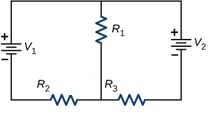We need at least 9 more requests to produce the answer.

1 / 10 have requested this problem solution

The more requests, the faster the answer.

All students who have requested the answer will be notified once they are available.

#### Earn Coins

Coins can be redeemed for fabulous gifts.

Similar Homework Help Questions
• ### Three resistors, R1 2.0 Ω, R2:4.0 Ω, and R3:6.0 Ω are connected as shown in the...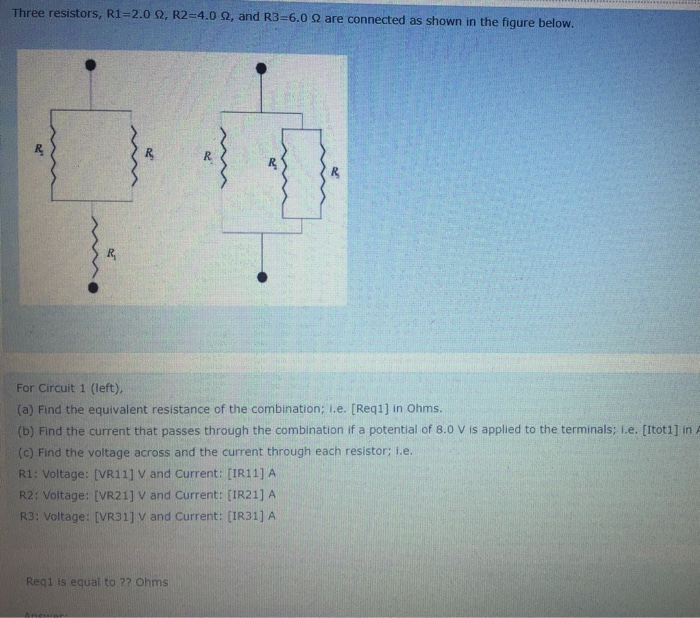Three resistors, R1 2.0 Ω, R2:4.0 Ω, and R3:6.0 Ω are connected as shown in the figure below. For Circuit 1 (left), (a) Find the equivalent resistance of the combination; .e. [Req1] in Ohms. (b) Find the current that passes through the combination if a potential of 8.0 V is applied to the terminals: L.e. [Itot1] in A (c) Find the voltage across and the current through each resistor; L.e R1: Voltage: [VR11] V and Current: [IR11] A R2: Voltage:...

• ### Rc circuit R2 7) R3 Four resistors of values R1 = 2.0 Ω, R2 = 4.0...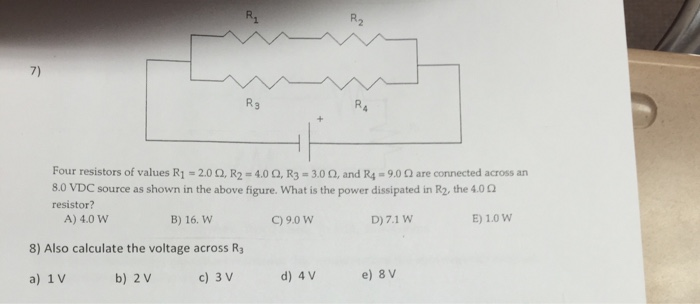Rc circuit R2 7) R3 Four resistors of values R1 = 2.0 Ω, R2 = 4.0 Ω, R3-3.00, and R4° 9.0 Ω are connected across an 8.0 VDC source as shown in the above figure, what is the power dissipated in R2, the 4.00 resistor? A) 4.0 W B) 16. W C)9.0 W D) 7.1 W E) 1.0 W 8) Also calculate the voltage across R a) 1 V b) 2 V c) 3 V d) 4 V

• ### The circuit shown in the figure below contains three resistors (R1, R2, and R3) and three...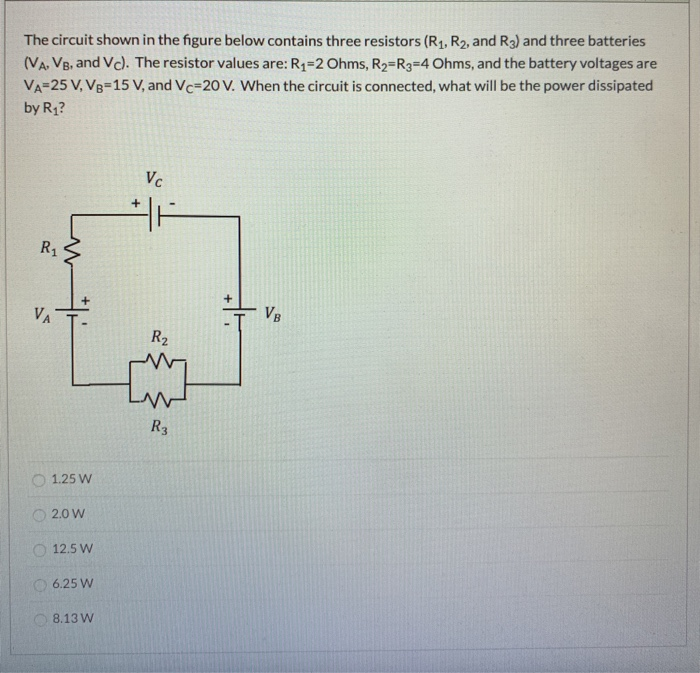The circuit shown in the figure below contains three resistors (R1, R2, and R3) and three batteries (VA, VB, and Vc). The resistor values are: R1=2 Ohms, R=R3=4 Ohms, and the battery voltages are VA=25 V, V8=15 V, and Vc=20 V. When the circuit is connected, what will be the power dissipated by R1? Vc M R1 + VA VB - R2 R3 1.25 W 2.0W 12.5 W 6.25 W 8.13 W The circuit shown in the figure contains four...

• ### Three resistors, R1-2.0 Ω, R2-4.0 Ω, and R3 6.0 Ω are connected as shown in the...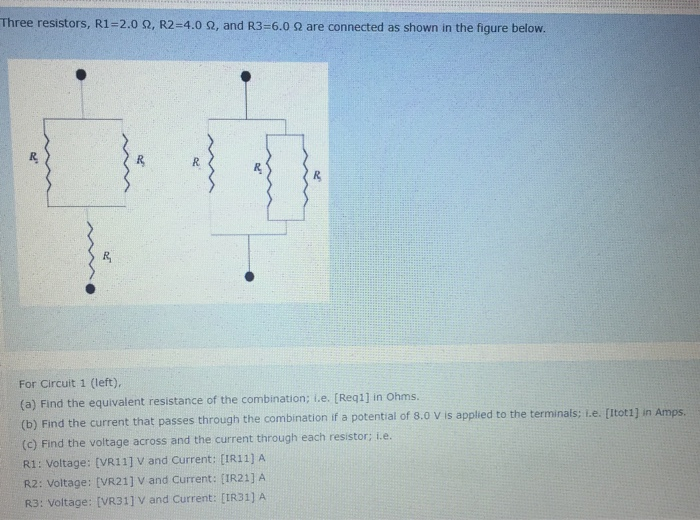Three resistors, R1-2.0 Ω, R2-4.0 Ω, and R3 6.0 Ω are connected as shown in the figure below. For Circuit 1 (left), (a) Find the equivalent resistance of the combination; t.e. Req1] in Ohms ) Find the current that passes through the combination if a potential of 8.0 V is applied to the terminals; L.e. [itot1) in Amps. (c) Find the voltage across and the current through each resistor; L.e. R1: Voltage: [VR11] V and Current: [IR11] A R2: Voltage:...

• ### The circuit shown in the figure below contains three resistors (R1, R2, and R3) and three...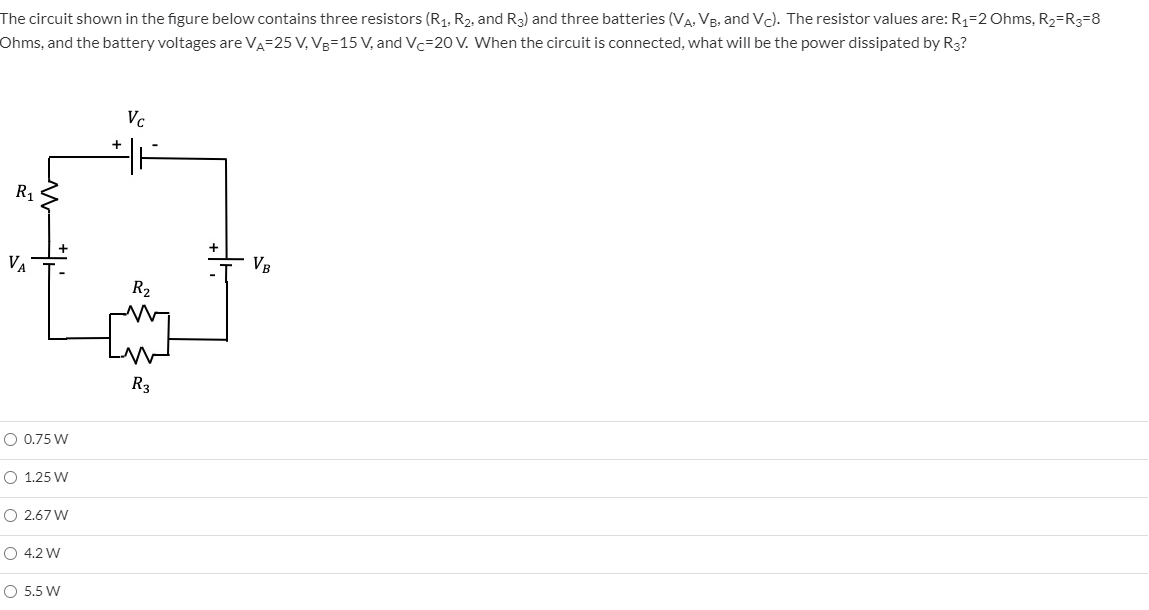The circuit shown in the figure below contains three resistors (R1, R2, and R3) and three batteries (VA, VB, and Vd). The resistor values are: R1=2 Ohms, R2=R3=8 Ohms, and the battery voltages are VA=25 V, V8=15 V, and Ve=20 V. When the circuit is connected, what will be the power dissipated by R3? Vc R1 VA V. R2 R3 O 0.75 W O 1.25 W 0 2.67 w 4.2 w O 5.5 W The circuit shown in the figure...

• ### Consider a circuit with four resistors in series. R1 R2 R3 R4 The battery has a...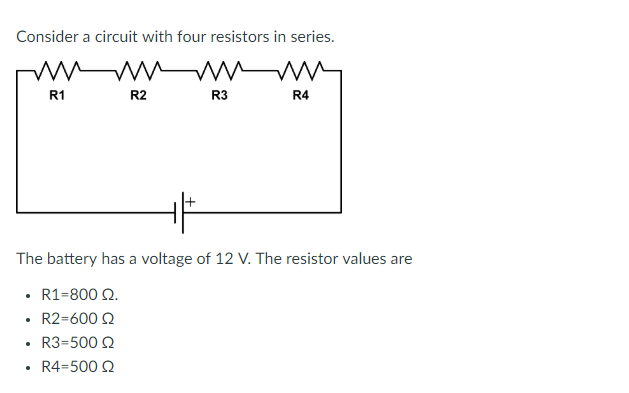Consider a circuit with four resistors in series. R1 R2 R3 R4 The battery has a voltage of 12 V. The resistor values are R1-800 Ω. R2-600 Ω R3-500 Q . R4-500Q Complete the following table by noting the voltage drop across each listed resistor. Resistor (Q) Voltage Drop (V) R1 00Select R3500Select ] 00 Select ] R2 R4

• ### In the circuit below, R1 = 83 Ω, R2 = 116 Ω, R3 = 119 Ω,...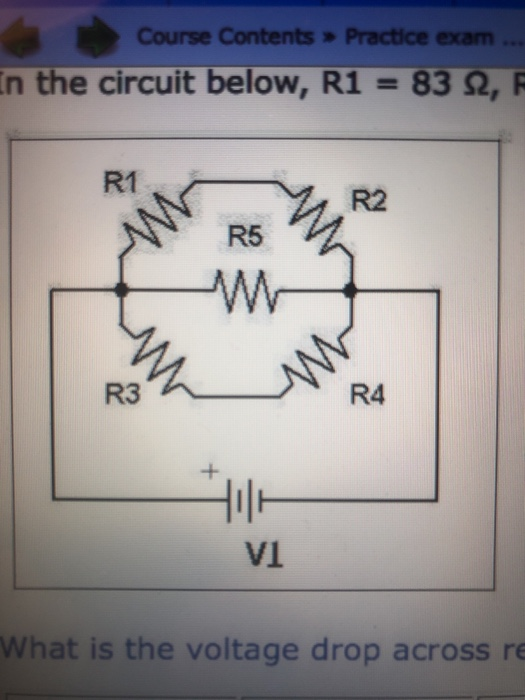In the circuit below, R1 = 83 Ω, R2 = 116 Ω, R3 = 119 Ω, R4 = 52 Ω, R5 = 247 Ω and the voltage supplied by the power supply is V1 = 76 V. 1. What is the voltage drop across resistor R2? (in V) 2. What is the voltage drop across restore R3? (in V) Course Contents > Practice exam ... In the circuit below, R1 = 83 2, F R2 R5 AAA WY R4 What...

• ### Consider the circuit shown in (Figure 1). The batteries have emfs of ε1 = 9.0 V and ε2 = 12.0 V and the resistors have values of R1 = 27Ω, R2 = 60 Ω, and R3 = 33 Ω.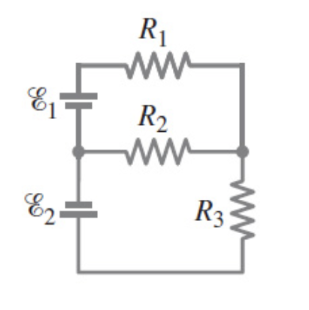Consider the circuit shown in (Figure 1). The batteries have emfs of ε1 = 9.0 V and ε2 = 12.0 V and the resistors have values of R1 = 27Ω, R2 = 60 Ω, and R3 = 33 Ω. Determine the magnitudes of the currents in each resistor shown in the figure. Ignore internal resistance of the batteries. Determine the directions of the currents in each resistor. Ignore internal resistance of the batteries.

• ### The circuit shown in the figure below contains three resistors (R1, R2, and R3) and three...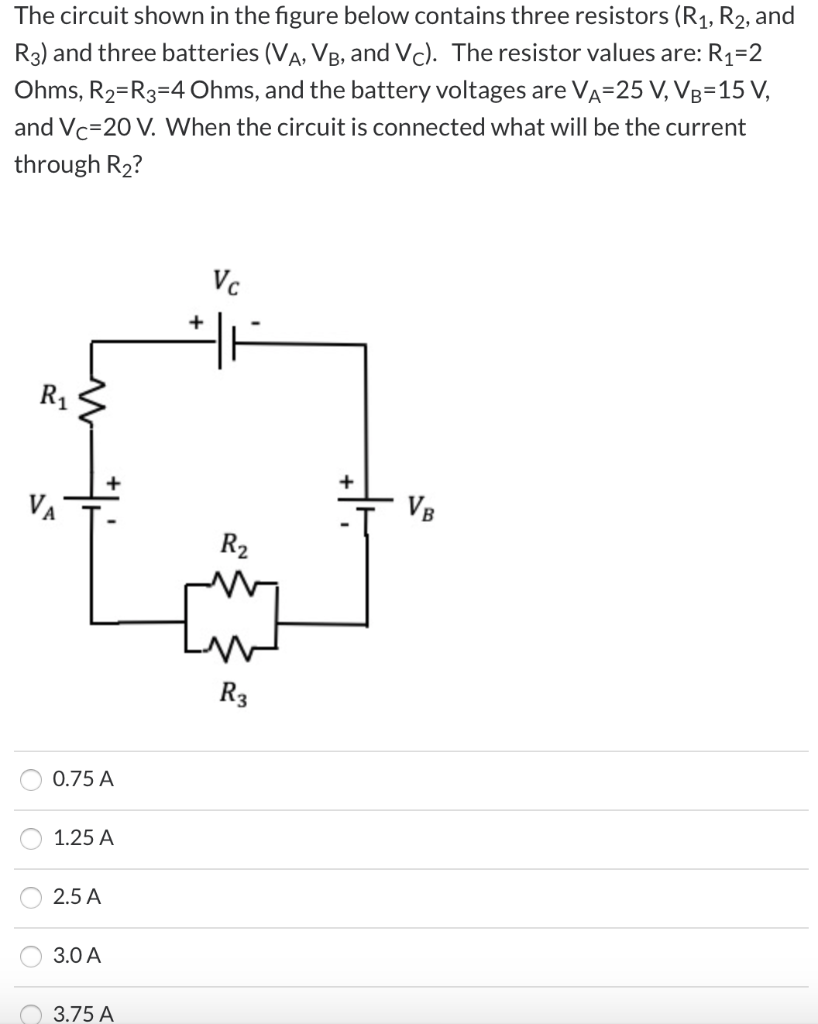The circuit shown in the figure below contains three resistors (R1, R2, and R3) and three batteries (VA, VB, and Vc). The resistor values are: R1=2 Ohms, R2=R3=4 Ohms, and the battery voltages are Va=25 V, V8=15 V, and Vc=20 V. When the circuit is connected what will be the current through R2? Vc R1 + VB R2 R3 0.75 A 1.25 A 2.5 A 3.0 A 3.75 A

• ### The circuit shown in the figure below contains three resistors (R1, R2, and R3) and three...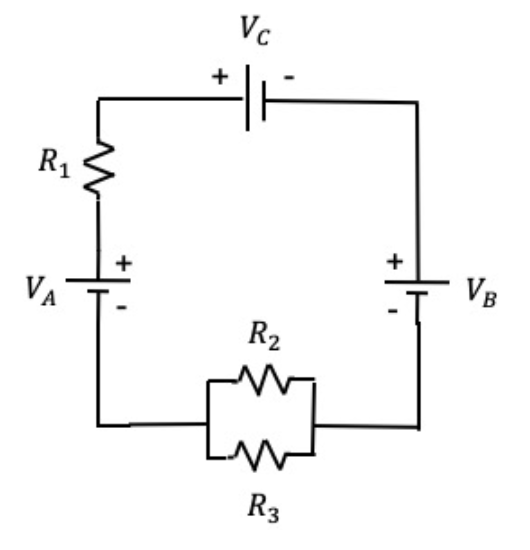The circuit shown in the figure below contains three resistors (R1, R2, and R3) and three batteries (VA, VB, and VC). The resistor values are: R1=2 Ohms, R2=R3=4 Ohms, and the battery voltages are VA=25 V, VB=15 V, and VC=20 V. When the circuit is connected, what will be the power dissipated by R3? options: 1.25 W 2.0 W 5.0 W 6.25 W 8.13 W Vc + R1 + + VA VB R2 R3